Kirchhoff method

(diff) ← Older revision | Latest revision (diff) | Newer revision → (diff)
A method for approximately solving problems in the theory of the diffraction of short waves; proposed by G.R. Kirchhoff. In its simplest version Kirchhoff's method amounts to the following: Let a wave process be described by the Helmholtz equation and consider the problem of the scattering of a plane wave by a convex surface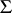on which the classical (Dirichlet) boundary conditionholds. The solution reduces to finding a functionsatisfying the Helmholtz equation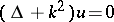subject to the indicated boundary condition and representable as the sum, wheresatisfies the Sommerfeld radiation conditions. The solution of the problem exists and it has the integral representation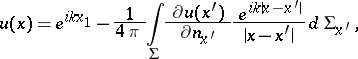(1)where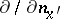is the derivative along the normal to. The normal is taken outward relative to the infinite domain bounded internally by. It is assumed that on the part ofilluminated by the plane wave,is approximately equal to the expression obtained by the ray method. On the shadowed part one sets. The expression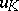obtained in this way is called the Kirchhoff approximation for.
In the illuminated region,and the geometric approximation forare the same in their principal terms. In a neighbourhood of the boundary between the illuminated and shadowed zones, the principal term of the asymptotic expansion ofis expressed in terms of the Fresnel integral, and in the shadowed zone(in fact in the shadowed zonedecreases considerably faster than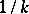).
The Kirchhoff method gives a formula forthat is correct in the principal terms and remains correct as. In the subsequent orders inthe Kirchhoff approximation is no longer applicable.